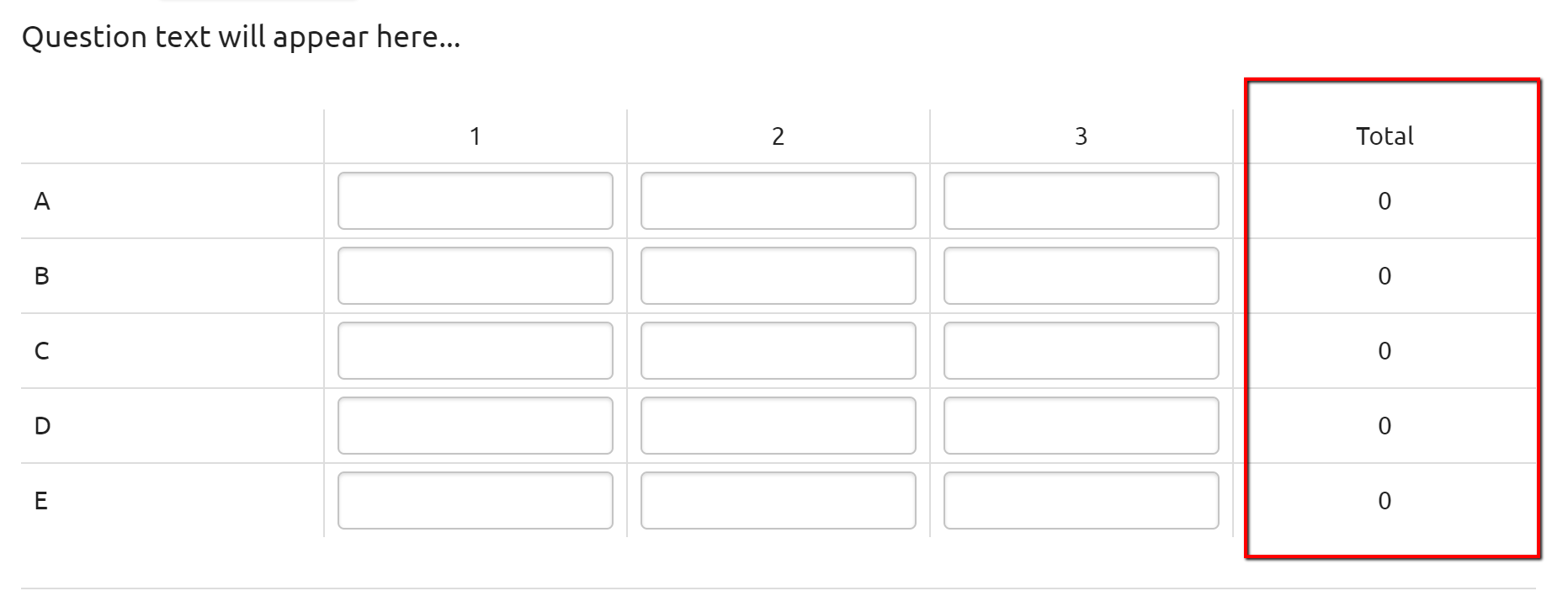# Script: Simple Grid > Horizontal Numeric - Running Total

This article will show you how to add a running total column for a Horizontal Numeric Simple Grid

### Background

By default Numeric Simple Grids do NOT have a built in Running Total option. This code will show you how to add a running total column for a Horizontal Numeric Simple Grid.### Instructions

1. Program your Horizontal Numeric Simple Grid
2. Program an additional column and title it as you see fit. This will be what is displayed to a respondent. In the example above we used the word "Total".
3. Add the following code to the Javascript field
```var \$ = jQuery;

/* Horizontal Grid */
\$(".cm-simple-grid__desktop").find("tr").each(function(idx) {
\$(this).find(".cm-numeric-input-container:last").append("<div class='total'></div>")
});

\$("input[type='number']").on("keyup", function(e) {
let \$myQuestionId = \$(this).closest(".cm-numeric-input-container").attr("questionid");
let total = 0;
let \$inputs = \$("[questionid='"+\$myQuestionId+"']").find("input[type='number']");
\$inputs.each(function(idx) {
if ((idx+1) < \$inputs.length) {
if (\$(this).val().length) total += parseInt(\$(this).val());
}
});
\$("[questionid='"+\$myQuestionId+"']").find(".total").text(total);
}).keyup();
```
4. Add the following code to the CSS field
```.cm-simple-grid__desktop > tbody > tr > .cm-simple-grid__cell:last-child > .cm-numeric-input-container input[type='number'] {
display: none;
}
```
5. Note:
1. This code is for display purposes only. These number will not be available to you in the data.
2. If you need to use these 'total' numbers, you will need to create either hidden questions or custom variables to store this data and set them with logic.